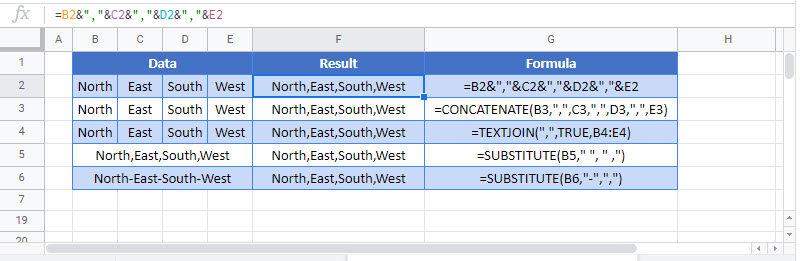This tutorial will demonstrate how to add commas in Excel and Google Sheets.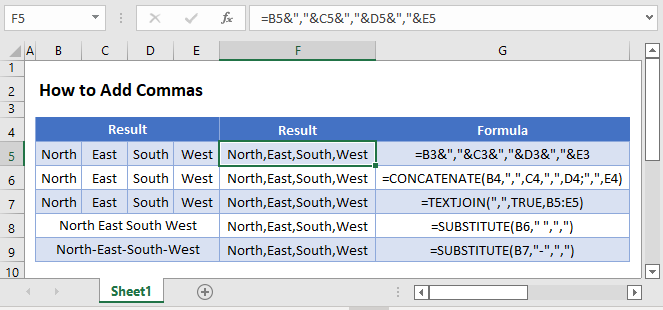To merge two cells together with a comma between the cell values, use this formula:

``=B3&","&C3``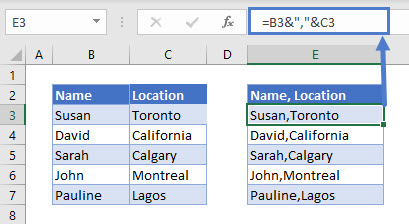In this example we’ve merged two cells together, separating the cell values with a comma. We use the ampersand operator (“&”) to merge the two cells and the comma together.

Note: When entering text into Excel formulas (in our case a comma), remember to surround the comma with quotations.

## Add Comma to a List (Excel 2019 and Later)

Commas can also be added to lists in Excel and Google Sheets with the use of the TEXTJOIN Function.

``=TEXTJOIN(",",TRUE,B3:B6)``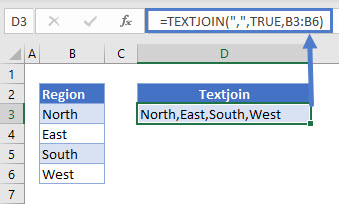The TEXTJOIN Function simply combines a range of cells together, separated by a delimiter (in our case a comma).

In the example above, within the TEXTJOIN Function we first enter the comma as the delimiter, next we choose to ignore blank cells by selecting “TRUE” and then we select the range to merge (“B3:B6”).

The TEXTJOIN Function was introduced in Excel 2019.

## Replace space by Comma

You can use the SUBSTITUTE Function to replace one character with another. Here we will replace all spaces (” “) with commas (” ,”)

``=SUBSTITUTE(B3," ", " ,")``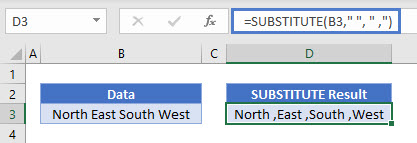Here we replace the dash (-) with commas.

``=SUBSTITUTE(B3,"-",",")``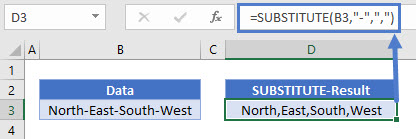## Create Running Lists with Commas

To create a running list with commas, use the following formula:

``=C3&","&B4``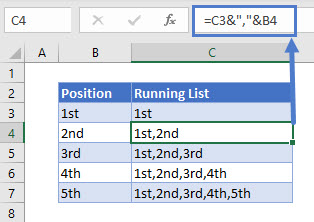The formula creates a list that keeps building for each row. Using the ampersand operator, the cell above is joined to the cell to the left.